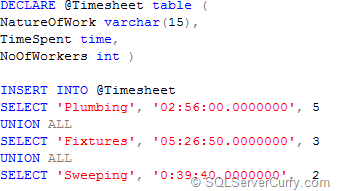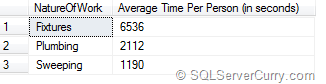### SQL Server 2008: Divide Time Column with Another Column

Here’s a simple example that divides a SQL Server 2008 Time column with another to calculate the time taken per employee to finish the task.

Let’s see a sample table firstDECLARE @Timesheet table (
NatureOfWork varchar(15),
TimeSpent time,
NoOfWorkers int )

INSERT INTO @Timesheet
SELECT 'Plumbing', '02:56:00.0000000', 5
UNION ALL
SELECT 'Fixtures', '05:26:50.0000000', 3
UNION ALL
SELECT 'Sweeping', '0:39:40.0000000',  2

In order to calculate the average time taken per worker to complete the task, we will need to divide the ‘TimeSpent’ column with the ‘No of Workers’ column. We will use the DATEDIFF function to return the time in seconds and then divide it by no. of workers to find the time taken by each worker to complete the task.
SELECT NatureOfWork,
SUM(DATEDIFF(SECOND, '00:00:00',TimeSpent))/
SUM(NoOfWorkers)
as [Average Time Per Person (in seconds)]
FROM @Timesheet
GROUP BY NatureOfWork

OUTPUT